## Friday 23 May 2014

### Gambling and Expected Value: Pick 2 and Pick 4 (OLG)

In this post on Gambling and Expected Value, we look at two more "Pick" lotteries, Pick 2 and Pick 4.
Click here to find similar posts on other lotteries and games of chance.

### Pick 2 (OLG)

Pick 2 is a lottery offered by the Ontario Lottery and Gaming Corporation (OLG).

### How the Game Works

The player chooses two numbers from '0' to '9'. Numbers can be repeated, so there are 100 possible selections. Players win if their selection is an exact match to the winning draw (i.e. match both numbers in the same order). A secondary prize is offered to players who match only the first digit (also in the same order).

### Probabilities and Prizes

The probability of matching both digits in the correct order is 1/100 (1%), with a prize of \$99 on a \$2 wager (\$49.50 per \$1 wagered). The probability of matching only the first digit is 9/100 (9%) and nets a prize of \$2 on a \$2 wager (\$1 per \$1 wagered). You might be wondering why it's a 9% chance of getting the smaller prize instead of 10%. There is a 1 in 10 chance (10%) of correctly matching the first number. However, that includes the probability of also matching the second number. We needed the probability of matching the first digit but not the second digit. In other words, the probability of matching the first number minus the probability of matching both: 10% - 1% = 9%.

Note: This game costs \$2 to play, but for analysis it's convenient to normalize prizes to dollars won per dollar wagered. It gives us a consistent basis to compare different lotteries or games that have varying minimum wagers.

### Example

Four gamblers, 'A', 'B', 'C', and 'D' play Pick 2.
'A' chooses 42.
'B' chooses 24.
'C' chooses 43.
'D' chooses 22.
All four of them bet \$2. The winning numbers drawn are 42.
'A' wins \$99.
'C' wins \$2.
'B' and 'D' lose.

### Expected Value

The expected value of Pick 2 is the weighted average value of the monetary gains or losses incurred from playing. That's pretty straightforward in Pick 2 because there are only three possible outcomes with only one way to play the game. Multiply the net gain from each outcome by the probability of that outcome, then sum for all outcomes. Keep in mind that the cost of playing must be subtracted from the winnings in order to calculate net gain. The expected value, expressed in dollars per dollar wagered is:
(48.50)×(1/100) + (0)×(9/100) + (-1)×(9/10) = -0.415
This means that, on average, you lose 41.5 cents for every dollar wagered playing Pick 2. Better for your bank account than playing the WCLC's version of Pick 3, analyzed previously, but still a fairly efficient way throw your money away.

### Pick 4 (OLG)

Pick 4 is also a lottery offered by the OLG.

### How the Game Works

Similar to Pick 2, the player chooses numbers from '0' to '9' (four choices this time instead of two). Numbers can be repeated, so there are 10,000 possible selections. There are two ways to play: Straight and Box. In Straight play, the order of the digits matters. The player must match the winning numbers in the same order in order to win.

In Box play, the order of the digits doesn't matter, just their values. 1334 is a match to 3341 in Box play, but not in Straight play. The benefit is that you increase your probability of winning in Box play, but the drawback is that the prize is reduced accordingly. Within Box play there are four possibilities, depending on the player's digit selections: 4-way, 6-way, 12-way, and 24-way. 4-way means there are four unique ways to arrange the four digits, which means there are only two unique digits (one of them is repeated twice; e.g. 1222). Likewise, 6-way, 12-way, and 24-way mean 6, 12, and 24 unique arrangements are possible. 6-way means there are two sets of digits that are the same (e.g. 1212). 12-way happens with three unique digits (one is repeated; e.g. 1231) and 24-way happens with four unique digits (e.g. 1234).

### Probabilities and Prizes

The various prizes available in Pick 4, and their respective probabilities, are summarized in the table below.

### Expected Value

So there are five ways to play Pick 4, but because of some rounding by the OLG, some choices are slightly worse than others. For Straight play, the expected value is:
(4999)×(1/10000) + (-1)×(9999/10000) = -0.50
But for 24-way Box play, the expected value is:
(199)×(0.0024) + (-1)×(0.9976) = -0.52
The table below shows the expected value for all five possible ways to play Pick 4.

From the expected value we can draw the following conclusions:
1. If you're going to play the lottery anyway, Pick 2 is a smarter choice (you'll lose money quicker in the long run playing Pick 4).
2. If you're going to play Pick 4, it's smarter to choose the Straight play option or the Box option with a 4-way combination.

## Thursday 22 May 2014

### Gambling and Expected Value: Pick 3 (WCLC)

Gamblers have many options available to them, but by and large the typical gambler knows little about how games of random chance work or how to assess the difference between various gambling options. This is the first of several posts to describe and analyze various ways to gamble. Here we'll be looking at the Pick 3 lottery offered by the Western Canada Lottery Corporation.

A simple metric to compare two different gambling options is the expected value. In a gambling scenario, the expected value of interest is the expected monetary loss. Comparing the expected value between two games lets you know which is a worse choice. Note: Because all games have a negative expected value, the smartest choice is always to not gamble at all.

Click here to find similar posts on other lotteries and games of chance.

### How the Game Works

The player chooses three numbers from '0' to '9' and the numbers can be repeated. Therefore, there are 10³ = 1,000 possible selections. From there, there are three ways to play the game: Straight, Box, and Straight/Box. In "straight" play the order of the digits matters. The player wins if his/her numbers are an exact match to the numbers drawn (i.e. chosen in the same order), but loses otherwise.

In "box" play the order of the digits doesn't matter. If the player's three digits come up in the winning numbers in any order, the player wins. If the player chose three unique digits there are 6 possible draws favourable to the player. If the player chose two unique digits there are 3 possible draws that would win. Obviously, box play isn't an option if all three digits are the same. If the player chose box play but happened to get the order correct, he/she still gets the smaller prize.

In "straight/box" play the player is choosing both straight and box methods simultaneously. The perceived benefit of straight/box play is that the player gets the improved probability of winning afforded by box play coupled with a chance at a larger prize if he/she happens to get the order correct. However, the prize for the correct order is smaller in straight/box play than in straight play. Similarly, the prize for the correct digits but wrong order is smaller in straight/box play than in box play.

### Probabilities & Prizes

In the table below, the possible prizes and their probabilities have been summarized for all possible ways to play Pick 3.

### Example

Five gamblers play Pick 3: 'A', 'B', 'C', 'D', and 'E'.
'A' chooses 123 and Straight.
'B' chooses 123 and Box.
'C' chooses 231 and Box.
'D' chooses 123 and Straight/Box.
'E' chooses 231 and Straight/Box.

They all bet \$1. The winning numbers drawn are 123. They are all winners, but with different prizes.
'A' wins \$500
'B' and 'C' each win \$80
'D' wins \$290
'E' wins \$40

### Expected Value

Calculating expected value is pretty straightforward. Take the weighted average of all possible outcomes of the game. We'll work through the Straight/Box type play for a 6-way combination. There are three possible outcomes with Straight/Box play: win with the exact order, win with the wrong order, or lose. Winning with the exact order occurs with a probability of 1 in 1000 and results in a net gain of 289 times the wager. You had to pay the money to play so your gain is your winnings less the wager. Winning with the wrong order occurs with a probability of 1 in 200 and results in a net gain of 39 times the wager. Losing occurs with a probability of 497/500 (99.4%) and results in a net loss of 1 times the wager. The probability of losing, i.e. the probability of not winning, is equal to:
(probability of straight win)  (probability of box win) = 100%  0.1%  0.5% = 99.4%
To calculate the weighted average outcome, multiply the net gain by its probability for each outcome and then sum for all outcomes. In this case:
289×0.001 + 39×0.005 + (-1)×0.994 = -0.51
What does that mean? It means that played over and over again, on average, you'd lose money at a rate of \$0.51 for every \$1 you ever wagered. Expected values for all types of play are given in the table below.

What do the numbers tell us? First, it tells us that the Pick 3 lottery is a pretty bad way to gamble as far as gambling goes. On average, you're losing more than half your wager every time you play. Second, all three ways to play have roughly the same expected value, so there isn't a big difference to your pocket in the long run. However, Box play is the worst choice by a small margin, followed by Straight/Box. If you're going to play Pick 3, you'll lose less money in the long run if you always choose the Straight play option.

## Friday 9 May 2014

### The Birthday Problem: Round 4

We've looked at the probability of any two people having the same birthday in a group. We've also looked at the probability of two people having a "near match" in a group. We then looked at the probability of the first match or near-match as people join a group one at a time. But what happens when we want to match (or nearly match) a specific birthday (your own for example).

Matches to your birthday will follow a binomial distribution, which has a probability mass function: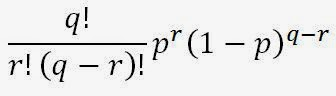Equation 1

where q is the number of trials, r is the number of successes, and p is the probability of success. If we're trying to match your birthday in a random group of people, r is any whole number greater than zero, p is (2k+1)/365 chance of an individual matching your birthday to within k days, and q is one less than the number of people in the group. If we substitute (n-1) = q, (2k+1)/365 = p, and r = 0 into Equation 1, we'll get the probability of NOT matching (or nearly matching) your birthday in a group of n people. That gives us Equation 2 below:Equation 2

Subtract from 100% to get the probability of at least one match to your birthday within k days among a total of n people. After simplifying, we get Equation 3:Equation 3
Plotting for different values of k and n (k = 0 if you're looking for a same-day birthday match) gives a graph like this:

It's hard to see the full picture with both k = 0 and k = 30 on the same plot, so here's another one:

We can also make a table showing the minimum size of the group for a corresponding probability that your birthday will be matched or nearly matched:

As you can see, matching your birthday is far less likely than matching any birthday. The results might be surprising though. Recall that we need only 23 people for there to be better than a 50% chance that two people in the group had matching birthdays. But in a group of you and 22 other people, there's only a 5.86% chance that your birthday is shared with someone else in the group. You need at least 253 other people in the group before it is more likely than not that your own birthday is shared with someone else.

We can also repeat the analysis from the third birthday post and figure out, if people entered randomly one at a time, who would be most likely to match your birthday. That perhaps isn't so interesting because the first person to enter after you always has the greatest chance of being the first person to match your birthday. The incremental probability of matching your birthday in a group of n versus n+1 people (i.e. the probability that the next person matches your birthday) is equal to: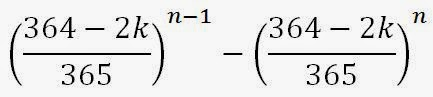Equation 4

It can be shown that Equation 4 is maximized by n = 1, irrespective of k. Therefore, the first random person joining you at the party is the most likely person to match your birthday, with a probability of a match simply equal to:Equation 5

For completion, here is the plot of equation 4 and the tabulated data to show the probability of the first person entering the group after you matching your birthday.

In short, matching a specific birthday in a random group of people is uncommon and generally requires a large sample, but it's not unusual for any two people in a small group of random people to share a birthday. And now there will be no more talk of birthdays for a long time.

## Friday 2 May 2014

### Another Variation of the Birthday Problem

In my first post on the Birthday Problem we determined the probability of there being a shared birthday in a group of n random people. In my second post, we expanded that to look at a more general problem of having a birthday within k days time of another birthday in a group of n random people.

This time, we'll be looking at the problem of determining which person in a list is most likely to share a birthday with the people before him. In other words, if randomly selected people enter a room one at a time, which person is most likely to be the first one to share a birthday with someone already in the room? Perhaps you'd like to know because you're looking for a gambling opportunity, betting your friends on which person arriving at a party will share a birthday with someone already there.

The probability of there being a shared birthday among a randomly selected group of n people can be calculated from the expression below:Equation 1

Suppose now we add one more person. The probability of there being a shared birthday in a group of n+1 people is:Equation 2

The incremental change in the probability of there being a shared birthday in a group of n people versus n+1 people is the probability that the (n+1)th person is the first person to share a birthday with someone already in the group. So subtracting the first expression from the second gives us the probability that the (n+1)th person added to a group of n people will share a birthday with someone already.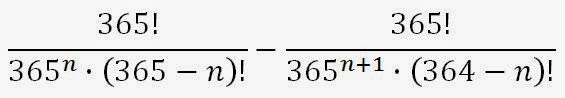Equation 3

Now if we want to know what value of n maximizes this probability, we can plot the function to find out. I've done that here:

The peak is at n = 19. So, at 3.23%, the 20th person to enter a random group of people has the greatest chance of being the first one to share a birthday with someone already in the group.

Suppose now we repeat this analysis for the more general case of birthdays within k days time of each other. The probability of there being birthdays with k days of each other among a group of n people is: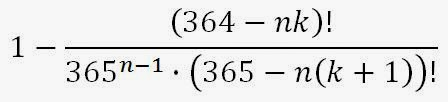Equation 4

And in a group of n+1 people, the probability is: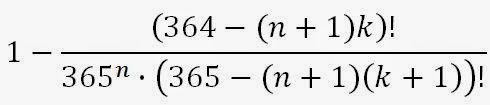Equation 5

Just as before, we can subtract Equation 4 from Equation 5 to get the probability that the (n+1)th person is the first person with a birthday close to one already in the group:Equation 6

Plotting this beastly looking function will allow us to see which person entering the room is most likely to be the one having a birthday close to a birthday of a person already at the party.

The table below summarizes the information from all the peaks in the above figure.

There you have it. As random people enter a room, the 3rd is most likely to be the first to have a birthday within a month of a person already at the party. The 6th is most likely to be the first to have a birthday within a week. The 12th person is most likely to have a birthday within one day, and the 20th is most likely to have the same birthday as someone already at the party.